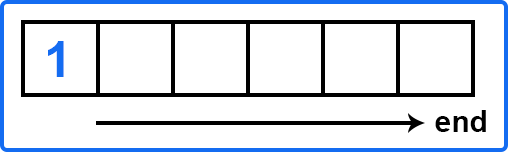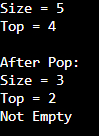# Stack in C++

## Stack in C++ with Examples:

In this article, I am going to discuss Stack in C++ with Examples. Please read our previous article, where we discussed Deque Class Functions in C++ with Examples.

##### Stack in C++ with Examples:

In the last article, we had seen about deque container that provided push and pop from both ends of the container. Here, the stack container adapter will adapt to the underlying container. In the case of a stack, by default, the underlying container will be deque.

In deque, we had both the facility insert and remove from both ends. So, what will stack do? It will act as a wrapper on that and it will hide some of those functionalities and expose only a few of them. For example, if we are allowed to insert and remove from both ends, this class wrapper will remove these facilities from one end. So first inserted element came to the left side,So, what it will do? It will convert the pushback in the end. It will expose push-back functionality as push and pop back as pop and hide the remaining two i.e. push front and pop front. This is what the container adapter is doing. Here, the intent is to provide the functionality of a stack to the programmers.

What is a Stack? Stack follows the last in first out principle which is LIFO. If we have a stack of plates, you keep a plate on top of the other plates, and when you remove it from the top, that was the one that was inserted in the end. Again, to insert, you will insert on the top and when you remove, you will remove from the top. So, whatever is last, is the one that is first picked out. This is LIFO (last in first out).

Similar to other STL containers, you need to include the stack header file ‘include <stack>’ in order to use these functionalities. And as explained earlier that we will call the top of the stack an end. In the deque, these were front and back, here on one end you cannot do anything. You are only working with one end and we will call this end the top of the stack.

##### Methods of Stack Container in C++

Now let us see some of the main functionalities of this container adapter,

1. size() – it is used to get the number of items present in a stack at a particular instant.
2. = – you can assign values or you can assign a new stack to it.
3. top() – it will access the top element of the stack.
4. empty() – empty checks whether there is any element or not in the stack.
5. push(), pop() – these are equivalent to push back and pop back. Now let’s write a program where we will use all these functions.
##### Example to Understand Stack in C++:
```#include <iostream>
#include <stack>
using namespace std;
int main()
{
// Create a stack of type integer.
stack<int> s;

// Insert the elements from 1 to 5 in the stack.
for(int i = 0; i < 5; i++){
s.push(i);
}

cout << "Size = " << s.size() << endl;  // Print the size of the stack.
cout << "Top = " << s.top() << endl;    // Print the top element of the stack.
cout << endl;

// Remove the elements from the stack (from the end).
s.pop();
s.pop();

cout << "After Pop: " <<endl;
cout << "Size = " << s.size() << endl;
cout << "Top = " << s.top() << endl;

if(s.empty())
cout << "Stack is Empty" <<endl;
else
cout << "Not Empty" << endl;

return 0;
}
```
###### Output: